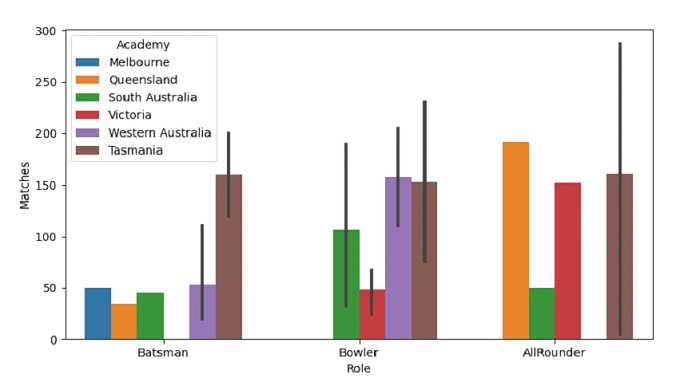Python Pandas - Draw vertical bar plots with nested grouping by two categorical variables in Seaborn

PythonServer Side ProgrammingProgramming

Bar Plot in Seaborn is used to show point estimates and confidence intervals as rectangular bars. The seaborn.barplot() is used for this. Plotting vertical bar plots grouped by a categorical variable, by passing categorical variables using x, y or hue parameter.

Let’s say the following is our dataset in the form of a CSV file − Cricketers2.csv

At first, import the required libraries −

import seaborn as sb
import pandas as pd
import matplotlib.pyplot as plt

Load data from a CSV file into a Pandas DataFrame −

Plotting vertical bar plots grouped by two categorical variables. The hue parameter also set

sb.barplot(x = dataFrame["Role"], y = dataFrame["Matches"], hue = "Academy", data= dataFrame)

Example

Following is the code −

import seaborn as sb
import pandas as pd
import matplotlib.pyplot as plt

# Load data from a CSV file into a Pandas DataFrame

# plotting vertical bar plots grouped by two categorical variables
# hue parameter also set
sb.barplot(x = dataFrame["Role"], y = dataFrame["Matches"], hue = "Academy", data= dataFrame)

# display
plt.show()

Output

This will produce the following output −